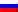# LP: Graphic resolution

Contenus

## Graphics resolution

It is possible to solve the problems having two variables (or two constraints for a dual problem) directly by a graphic resolution. The resolution process takes place in three stages:

1. Achievable domain
2. Plot the objective function
3. Determine the optimal solution

## Achievable domain

For that, we represent each constraint in the graph, by hatching or coloring the side which does not satisfy the constraint.
Thus, we highlight a domain of definition or realizable domain, any point of the domain of definition satisfies all the constraints of the mathematical model.

Consider the following linear program:

Let us draw the domain of definition:

Adding the first constraint and the type of variables

## Plot the objective function

In order to solve the problem, we represent the objective function at point (100,0) then at various points (following the gradient of the objective function) until the objective function has only one point or one facet of the definition field.

The gradient of the objective function is (350,300). The z value therefore increases when the objective function is moved in the same direction as the vector (350,300), therefore by moving towards the northeast corner. We can clearly see in the following figure that the value of z has increased by taking another line of the objective function.

We then obtain the optimal overall solution (s). If we trace the objective function again by following the gradient, the line will be outside the domain of definition.

## Optimal solutions

The optimal solution (s) are the last point (s) before the line of the objective function leaves the domain of definition.

The optimal solution is found at the intersection of the first and second constraint, therefore satisfies both constraints. The solution vector is the solution of the system:

The solution of this system is (122,78) which gives z = 66100.

## The four optimal solution possibilities

There are four possibilities:

• either a unique solution exists (a point);
• either an infinity of solutions (one facet);
• either the solution is not bounded, the line of the objective function will always be in the domain of definition by following the gradient;
• or there is no solution, for example if the domain is empty.FRFRENRUES